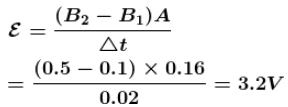Courses

# Electromagnetic Induction NAT Level - 1

## 10 Questions MCQ Test Topic wise Tests for IIT JAM Physics | Electromagnetic Induction NAT Level - 1

Description
This mock test of Electromagnetic Induction NAT Level - 1 for IIT JAM helps you for every IIT JAM entrance exam. This contains 10 Multiple Choice Questions for IIT JAM Electromagnetic Induction NAT Level - 1 (mcq) to study with solutions a complete question bank. The solved questions answers in this Electromagnetic Induction NAT Level - 1 quiz give you a good mix of easy questions and tough questions. IIT JAM students definitely take this Electromagnetic Induction NAT Level - 1 exercise for a better result in the exam. You can find other Electromagnetic Induction NAT Level - 1 extra questions, long questions & short questions for IIT JAM on EduRev as well by searching above.
*Answer can only contain numeric values
QUESTION: 1

### On passing a current of 2A in a coil of inductance 5H, the energy (in Joule) stored in it will be :

Solution: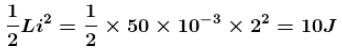*Answer can only contain numeric values
QUESTION: 2

### The self inductances of two identical coils are 0.1 H. They are wound over each other. Mutual inductance will be (in Henry).

Solution: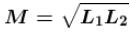*Answer can only contain numeric values
QUESTION: 3

### In the figure magnetic energy (in Joules) stored in the coil is (in steady state).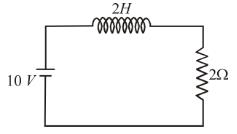Solution: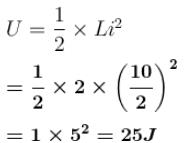*Answer can only contain numeric values
QUESTION: 4

When current flowing in a coil changes from 3A to 2A in the millisecond, 5 volt emf is induced in it. The self-inductance of the coil (in mH) will be :

Solution: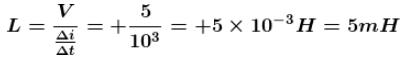*Answer can only contain numeric values
QUESTION: 5

The magnetic flux linked with the coil varies with time as  φ = 3t2 + 4t + 9. The magnitude of induced emf (in Volts) at  t = 2 second is.

Solution: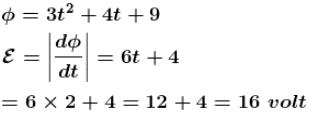*Answer can only contain numeric values
QUESTION: 6

The spokes of a wheel are made of metal and their lengths are of one metre. One rotating the wheel about its own axis in a uniform magnetic field of 5 × 10–6 tesla  normal to the plane of the wheel a potential difference of 3.14 mV  is generated between the rim and the axis. The rotational velocity  of the wheel is (in  rev/sec).

Solution: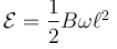*Answer can only contain numeric values
QUESTION: 7

If in a coil rate of change of area is 5m2/ms  and current becomes Amp from Amp in 2×10–3sec. If magnetic field is tesla  then self inductance (in Henry) of the coil is :

Solution: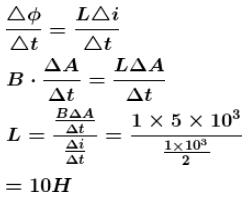*Answer can only contain numeric values
QUESTION: 8

When the primary current in the spark coil of a car changes from 4A to zero in 10µs, an emf of 40,000V  is induced in the secondary. The mutual inductance (in  Henry) between the primary and the secondary windings of the spark coil will be.

Solution: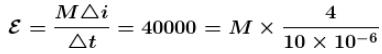M = 0.1H

*Answer can only contain numeric values
QUESTION: 9

A metallic rod completes its circuit as shown in the figure. The circuit is normal to a magnetic field  B = 0.15 tesla. If the resistances of the rod is  the force required to move the rod with a constant velocity of 2 m/sec is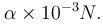Find the value of  α.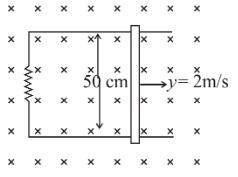Solution: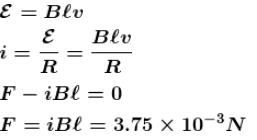*Answer can only contain numeric values
QUESTION: 10

Area of a coil is 0.16 m2. If magnetic field through it changes from 0.1 Wb/m2 to 0.5 Wb/m2  in  0.02 s, then the emf induced (in Volts) in the coil will be.

Solution: